#### Closed-form solutions

According to (14.1), the action trajectory must be integrated to produce a state trajectory. In some cases, this integration leads to a closed-form expression. For example, if the system is a chain of integrators, then a polynomial expression can easily be obtained for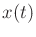. For example, suppose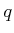is a scalar and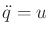. If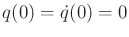and a constant action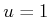is applied, then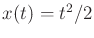. If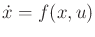is a linear system (which includes chains of integrators; recall the definition from Section 13.2.2), then a closed-form expression for the state trajectory can always be obtained. This is based on matrix exponentials and is given in many control theory texts (e.g, ).

Steven M LaValle 2020-08-14